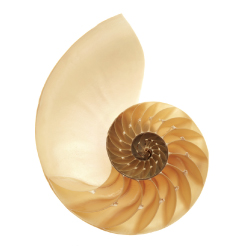### A Mathematical Concept — and a Glimpse of God

#### By Robbin O'Leary, Professor of MathematicsThe word "infinite" comes from Latin in (meaning "not") and finis (meaning "end"). Scripture does not actually use the word "infinite," but refers to God as being great beyond our comprehension, eternal, or without end in time. So the word "infinite" used in reference to God means that some aspect of God's character is without limit.

I come to this topic as a mathematician, not as a philosopher or a theologian. From my perspective, mathematical "sets" — specifically infinite sets — can provide a small insight into the nature of God.

When relating the language of infinity to God, we are using an analogy. The mathematical concept of infinity is very focused, and even though we sometimes don't understand mathematical infinity very well, we know much more about it than we do about God.

Stated simply, a set is a collection of objects. These objects might be words, such as the number words {one, two, three}. Two sets have the same number of elements if they can be put into one-to-one correspondence. This means they can be paired up with each other so that nothing is missed, and nothing is counted (or paired up) more than once. All sets have the same number of elements as each other have the same name: the last number word we reach when counting the elements. This is called the "cardinal number" of the set. For example, if I count the number of words in the set {one, two, three}, the last number I say, three, is the cardinal number of that set. Any set that can be counted in this way, where you end at some number, is called a finite set.

What if I have a set for which no such stopping point exists, say the set of all counting or natural numbers, ℕ? If we try to count all these numbers, we never finish. This set is not finite, so we say it is infinite.

This is an indirect method of defining a concept — by defining what it is not. So to say a set is infinite is to say that it is "not finite." Indeed, some of our descriptions of God also speak about what God is not — immortal (not mortal), invisible (not visible), unchangeable (not changeable), and others.

Mathematics also has a direct definition for infinite sets. Think about the even numbers, {two, four, six, eight ...}. This set can be put into one-to-one correspondence with the natural numbers, by pairing any even number with half itself. We're essentially counting the even numbers and finding that there are the same number of them as there are natural numbers.

So we have two sets, evens and natural numbers that are the same size, but the evens are a smaller set than the natural numbers because the set of evens fits inside the naturals with some numbers left over. (This is called a proper subset.) This property gives us a direct definition of an infinite set: a set that can be put into one-to-one correspondence with a proper subset of itself.

Looking at infinite sets in this direct way allows us to see some of the ways infinite sets are different from finite sets. Consider the simple act of subtracting. If you subtract with finite sets (think "take away"), you always get a smaller set. However, with the infinite set ℕ, taking away the odd numbers leaves the even numbers, another infinite set. Or, you can take the infinite set of all numbers greater than one from the infinite set ℕ and get a finite set, {one}. In other words, infinity minus infinity could be zero; it could be one; it could be infinity.

Infinite sets simply don't follow the same rules as finite sets. Subtracting a finite set from an infinite set leaves a set the same size as before, infinitely large. Any finite set is negligible — it might as well be nothing — in comparison to the unimaginable size of an infinite set.

By analogy, as God allows us to make choices, giving up some power, God remains all-powerful. In mathematics, we are very careful when discussing the infinite, because we know that infinite sets don't behave like finite sets. Our mathematical intuition built on our experience with the finite does not apply to the infinite.

Sometimes we Christians need to recognize with similar humility how much we don't understand God's ways. In our thinking we must allow God to be God, to be beyond our understanding, much as mathematicians allow the infinite sets of mathematics to be different from the finite.

Robbin O'Leary is a professor of mathematics at SPU.